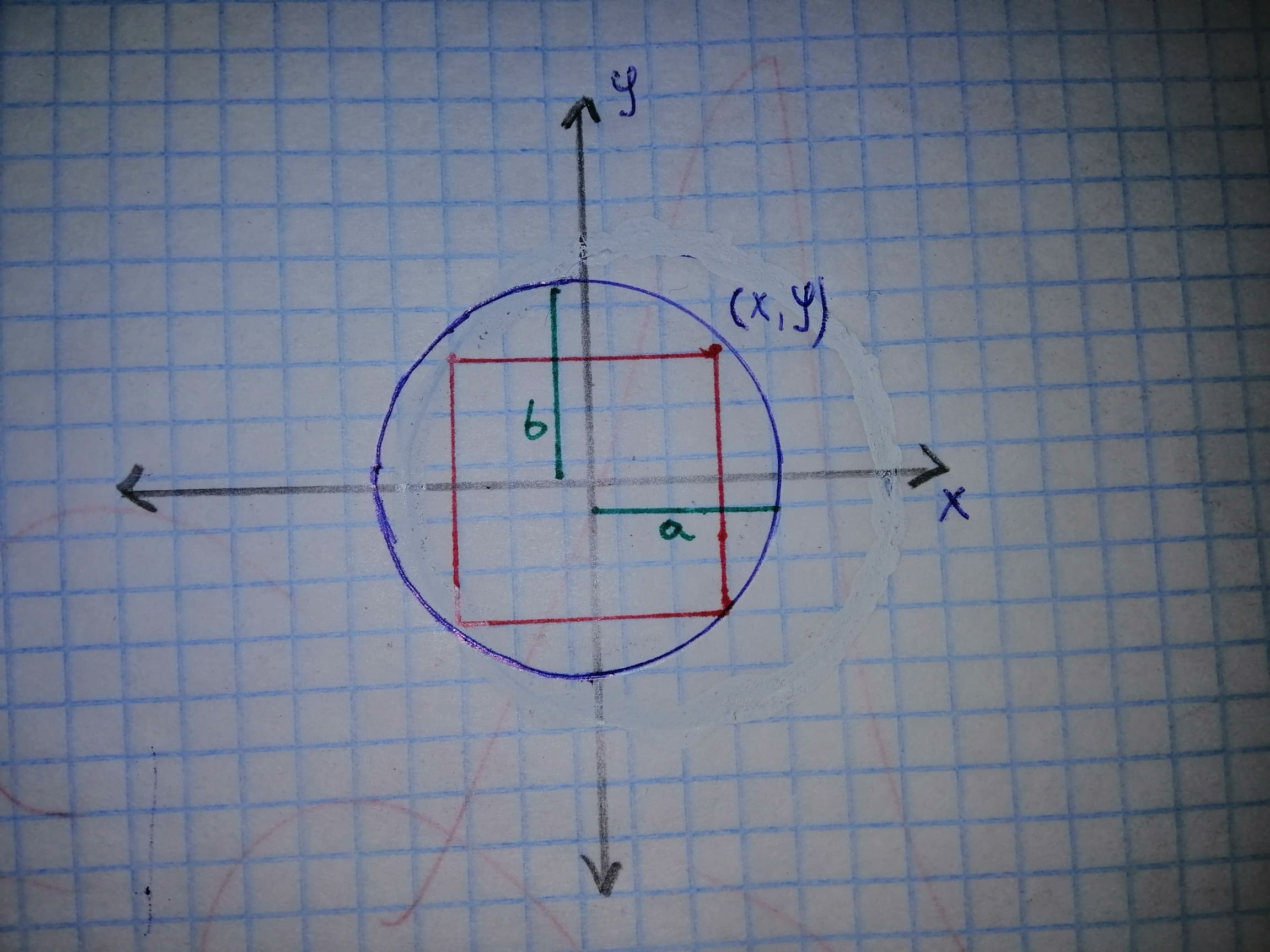# Prove these examples are correct: a) What is the area of the largest rectangle that fits inside of the ellipse x^{2} + 2y^{2} = 1? b) Prove the following: Let c in (a, b). If f is continuous on [a, b], differentiable on (a, b)?Elleanor Mckenzie 2021-02-25 Answered
Prove these examples are correct:
a) What is the area of the largest rectangle that fits inside of the ellipse

b) Prove the following: Let c in (a, b). If f is continuous on differentiable on (a, b)?
You can still ask an expert for help

• Questions are typically answered in as fast as 30 minutes

Solve your problem for the price of one coffee

• Math expert for every subject
• Pay only if we can solve itVelsenw

a) Сonsider this figure:From the figure it can be seen that:
Area $\left(A\right)=4xy$
And also,

We've taken the positive value since we chose this point to be in the first quadrant
So now deciding:
$A=4xy$

Differentiating the above function with respect to "y":

For maximize the area:
Put,
$\frac{dA}{dy}=0$

${y}^{2}=\frac{1}{8}$
$y=\frac{1}{\sqrt{8}}$
Corresponding to this,

$x=\sqrt{\frac{3}{4}}$
$x=\frac{\sqrt{3}}{2}$
Hence the maximum area:
Area $\left(A\right)=4xy$

$Are{a}_{max}=\sqrt{\frac{3}{2}}$
b)Prove the following: Let c in (a, b). If f is continuous on [a, b], differentiable on (a, b), and:

Properties used

Proof is given below:
Since:

By using the property: